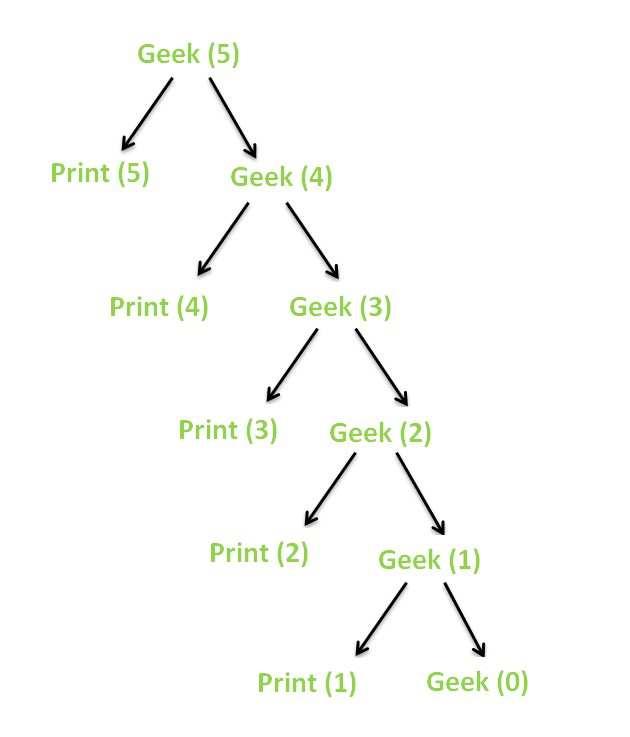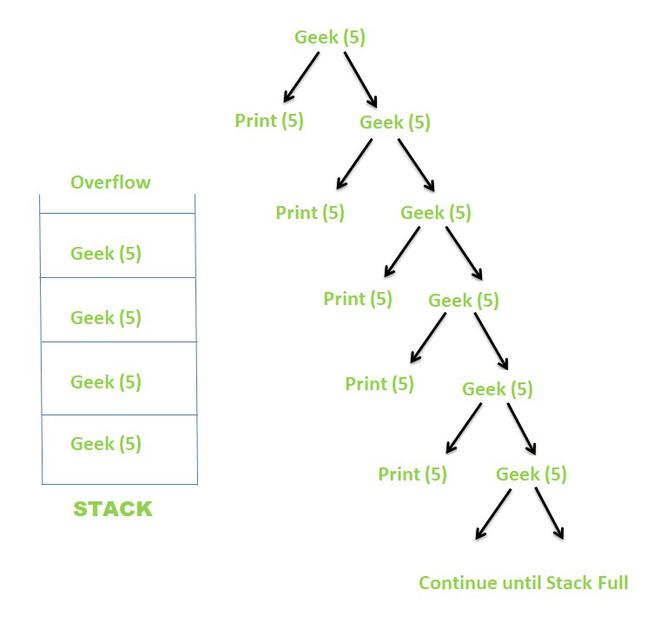Related Articles

# Finite and Infinite Recursion with examples

• Last Updated : 23 Jul, 2021

The process in which a function calls itself directly or indirectly is called Recursion and the corresponding function is called a Recursive function
Using Recursion, certain problems can be solved quite easily. Examples of such problems are Towers of Hanoi (TOH), Inorder/Preorder/Postorder Tree Traversals, DFS, etc.

Types of Recursions:

Take a step-up from those "Hello World" programs. Learn to implement data structures like Heap, Stacks, Linked List and many more! Check out our Data Structures in C course to start learning today.

Recursion can be further classified into two kinds, depending on when they terminate:

1. Finite Recursion
2. Infinite Recursion

Finite Recursion:

Finite Recursion occurs when the recursion terminates after a finite number of recursive calls. A recursion terminates only when a base condition is met.

Example:

Below is an implementation to demonstrate Finite Recursion.

## C++

 `// C++ program to demsonstrate Finite Recursion``#include ``using` `namespace` `std;` `// Recursive function``void` `Geek(``int` `N)``{``    ``// Base condition``    ``// When this condition is met,``    ``// the recursion terminates``    ``if` `(N == 0)``        ``return``;` `    ``// Print the current value of N``    ``cout << N << ``" "``;` `    ``// Call itself recursively``    ``Geek(N - 1);``}` `// Driver code``int` `main()``{` `    ``// Initial value of N``    ``int` `N = 5;` `    ``// Call the recursive function``    ``Geek(N);``    ``return` `0;``}`

## Java

 `// Java program for the above approach``class` `GFG{` `// Recursive function``static` `void` `Geek(``int` `N)``{``    ` `    ``// Base condition``    ``// When this condition is met,``    ``// the recursion terminates``    ``if` `(N == ``0``)``        ``return``;` `    ``// Print the current value of N``    ``System.out.println(N + ``" "``);` `    ``// Call itself recursively``    ``Geek(N - ``1``);``}` `// Driver code``public` `static` `void` `main(String[] args)``{``    ` `    ``// Initial value of N``    ``int` `N = ``5``;` `    ``// Call the recursive function``    ``Geek(N);``}``}` `// This code is contributed by abhinavjain194`

## Python3

 `# Python program to demsonstrate Finite Recursion``# Recursive function``def` `Geek( N):` `    ``# Base condition``    ``# When this condition is met,``    ``# the recursion terminates``    ``if` `(N ``=``=` `0``):``        ``return` `    ``# Pr the current value of N``    ``print``( N, end ``=``" "` `)` `    ``# Call itself recursively``    ``Geek(N ``-` `1``)`  `# Driver code``# Initial value of N``N ``=` `5` `# Call the recursive function``Geek(N)` `# this code is contributed by shivanisinghss2110`

## C#

 `// C# program for the above approach``using` `System;``using` `System.Collections.Generic;` `class` `GFG{` `// Recursive function``static` `void` `Geek(``int` `N)``{``     ` `    ``// Base condition``    ``// When this condition is met,``    ``// the recursion terminates``    ``if` `(N == 0)``        ``return``;`` ` `    ``// Print the current value of N``    ``Console.Write(N + ``" "``);`` ` `    ``// Call itself recursively``    ``Geek(N - 1);``}` `// Driver Code``public` `static` `void` `Main(String[] args)``{``  ` `    ``// Initial value of N``    ``int` `N = 5;`` ` `    ``// Call the recursive function``    ``Geek(N);``}``}` `// This code is contributed by target_2.`

## Javascript

 ``
Output
`5 4 3 2 1 `

The recursion tree for the above recursive function looks like this.Recursion Tree

When the value of N becomes 0, because of the base condition, the recursion terminates.

Infinite Recursion:

Infinite Recursion occurs when the recursion does not terminate after a finite number of recursive calls. As the base condition is never met, the recursion carries on infinitely.

Example:

Below is an implementation to demonstrate Infinite Recursion.

## C++

 `// C++ program to demsonstrate Infinite Recursion``#include ``using` `namespace` `std;` `// Recursive function``void` `Geek(``int` `N)``{``    ``// Base condition``    ``// This condition is never met here``    ``if` `(N == 0)``        ``return``;` `    ``// Print the current value of N``    ``cout << N << ``" "``;` `    ``// Call itself recursively``    ``Geek(N);``}` `// Driver code``int` `main()``{` `    ``// Initial value of N``    ``int` `N = 5;` `    ``// Call the recursive function``    ``Geek(N);``    ``return` `0;``}`

## Java

 `// Java program to demsonstrate Infinite Recursion``import` `java.io.*;`` ` `class` `GFG``{``// Recursive function``static` `void` `Geek(``int` `N)``{``    ``// Base condition``    ``// This condition is never met here``    ``if` `(N == ``0``)``        ``return``;` `    ``// Print the current value of N``    ``System.out.print( N +``" "``);` `    ``// Call itself recursively``    ``Geek(N);``}` `// Driver code``public` `static` `void` `main(String[] args)``    ``{` `    ``// Initial value of N``    ``int` `N = ``5``;` `    ``// Call the recursive function``    ``Geek(N);``    ``}``}` `// This code is contributed by shivanisinghss2110`

## Python3

 `# Python3 to demsonstrate Infinite Recursion` `# Recursive function``def` `Geek(N):``    ` `    ``# Base condition``    ``# This condition is never met here``    ``if` `(N ``=``=` `0``):``        ``return` `    ``# Print the current value of N``    ``print``(N, end ``=` `" "` `)` `    ``# Call itself recursively``    ``Geek(N)` `# Driver code` `# Initial value of N``N ``=` `5` `# Call the recursive function``Geek(N)` `# This code is contributed by shivanisinghss2110`

## C#

 `// C# program to demsonstrate Infinite Recursion``using` `System;`` ` `class` `GFG``{``// Recursive function``static` `void` `Geek(``int` `N)``{``    ``// Base condition``    ``// This condition is never met here``    ``if` `(N == 0)``        ``return``;` `    ``// Print the current value of N``    ``Console.Write( N +``" "``);` `    ``// Call itself recursively``    ``Geek(N);``}` `// Driver code``public` `static` `void` `Main(String[] args)``    ``{` `    ``// Initial value of N``    ``int` `N = 5;` `    ``// Call the recursive function``    ``Geek(N);``    ``}``}` `// This code is contributed by shivanisinghss2110`

## Javascript

 ``

The recursion tree for the above recursive function looks like this.Recursion Tree

Since the value of N never becomes 0, so the recursion never terminates. Instead, the recursion continues until the implicit stack becomes full which results in a Stack Overflow. Some compilers directly give the output as Segmentation Fault (Core Dumped), while others may abnormally terminate for some value and then show Segmentation fault

My Personal Notes arrow_drop_up